Trial ends in

# A Micro-Displacement Measurement Based on the Shadow Technique

Published: October 7, 2022 doi: 10.3791/64658

### Abstract

The precision measurement of micro displacement is important in scientific and industrial fields. However, it is a tough challenge due to the complex design and the high cost of measuring instruments. Inspired by the shadow formed from water striders walking on a water surface under sunlight, a micro-displacement measurement method was proposed. Water strider legs with superhydrophobic properties bend the water surface. The curved surface of the water refracts sunlight, creating a shadow with a bright edge at the bottom of the pond. The shadow size is generally larger than the indentation depth of the legs from the water surface. In the micro-displacement measurement system, the applied displacement is proportional to the change in the diameter of the shadow. The presented study proposes a micro-displacement measurement procedure based on this shadow technique. The displacement sensitivity can reach 10.0 nm/pixel in the range of 5 µm. This system is simple to construct, low cost, and has high precision with good linear performance. The method provides a convenient additional option to measure micro-displacement.

### Introduction

Precise displacement measurements play a vital role in the fields of aerospace engineering1, ultra-precision machining2, and micro-assembly3. Structural deformation must be measured precisely for structural health monitoring4. However, micro-displacement measurements with high precision remain a tough challenge due to the complex design and high cost of the measuring instruments5.

The micro-displacement measurement technique can be divided into conventional and non-conventional methods. Conventional methods, such as magnetic, capacitive, inductive, and electric sensors, are susceptible to electromagnetic interference6. Non-conventional methods are mainly optical methods, such as the optical fiber-based method and the laser method.

Ke Tian et al. designed a balloon-shaped bent multimode fiber structure to measure micro displacement, whose displacement sensitivity could achieve 0.51 dB/µm with a measuring range of 0-100 µm experimentally7. However, the size and the cost of the fiber optic demodulator must be considered first; and it was not easy to eliminate the thermal effect. Qianbo Lu et al. proposed a sub-nanometer resolution displacement sensor based on a grating interferometric cavity, whose sensitivity could reach 44.75 mV/nm by the intensity compensation and phase modulation8. The laser interferometer is one of the commonly used micro-displacement instruments with nanoscale resolution. However, the reflector requires complicated signal processing, and the fringe resolution of the interferometry limits its applications9. Therefore, an alternative simply-constructed, low-cost, high-precision measurement system is needed.

This article proposes a micro-displacement measurement procedure based on the shadow technique10,11,12,13 that is simple, low cost, and highly precise with good linear performance. This method was inspired by water striders walking on the water surface. Water strider legs with superhydrophobic properties bend the water surface. The curved surface of the water refracts sunlight, creating a shadow with a bright edge at the bottom of the pond. The shadow size is generally much larger than the indentation depth of legs from the water surface14,15,16. In the system, the applied displacement and the change in the diameter of the shadow were proportional, which was verified by the calibration experiment. The research indicates that this method provides an alternative for measuring micro displacement precisely.

### Protocol

1. Preparation of the PDMS piece

1. Prepare polydimethylsiloxane (PDMS, see Table of Materials) polymer by weighing the base and the curing agent (using a weighing balance) at a ratio of 30:1 in a cuboid polycarbonate (PC) container.
NOTE: The container size is 60 mm × 45 mm × 15 mm. The height of the mixture is about 10 mm.
2. Mix the PDMS mixture in a blender for about 20 min until it is filled with bubbles.
3. Use a vacuum pump to remove bubbles from the mixture for about 30 min (see Table of Materials).
4. Cure the PDMS at a 65 °C oven for 4 h.
5. Use the PDMS piece formed in the PC container as the displacement-sensitive element (step 2.1).
​NOTE: The PDMS piece size is 60 mm × 45 mm × 10 mm.

2. Experimental preparation for measuring micro-displacement

1. Gather all the modules/components of the measurement system, including a parallel light source, an aperture diaphragm having a 0.7 mm diameter, a piece of PDMS (the displacement-sensitive element), a workbench with three rigid cylindrical legs, a camera, a vertical precision positioner, motorized stages, and other components (see Table of Materials), as shown in Figure 1 and Figure 2.
2. Place the commercially obtained workbench (see Table of Materials) on the PDMS.
NOTE: The surface of the PDMS is bent by the legs of the workbench. The workbench is obtained by machining aluminum. The diameter of the rigid cylindrical legs is 0.5 mm, which needs to be as small as possible.
3. Connect the parallel light source with the aperture diaphragm to emit a parallel light beam with a certain diameter vertically to one of the rigid cylindrical legs.
NOTE: The diameter of the parallel light beam is 0.7 mm, which must be as small as possible.
4. Place the parallel light source about 50 mm above the PDMS surface by adjusting the motorized stages of the workbench.
NOTE: The bent surface refracts the parallel light creating a shadow with a bright edge, which is shown in Figure 3.
5. Place the camera directly at 260 mm under the PDMS to capture the image of the shadow. Adjust the magnification of the camera lens so that the shadow fills the entire image.
​NOTE: The camera's pixel size and resolution are about 3.72 µm and 4,000 × 6,000, respectively.
6. Fix the vertical precision positioner with a resolution of 0.2 nm above the workbench to calibrate the displacement sensitivity (step 4).

3. Image processing

1. Convert the color photograph of the shadow to a grayscale image using a machine vision software (see Table of Materials).
1. Click on the start button of the software to process the shadow image and obtain the shadow diameter directly.
NOTE: The code of image processing steps is integrated into the software.
2. Perform the preprocessing of the image, including Gaussian filtering to remove the noise and image enhancement to enhance image contrast12.
3. Use the Canny operator to calculate each pixel's gradient and obtain sub-pixel edge contour sets. Exclude non-edge pixels based on the non-maximum suppression12.
4. Eliminate the edge contours that are not part of shadow edges by setting a certain length threshold.
5. Fit the shadow diameter by the least-squares fitting of circles17.

4. Displacement calibration

1. Adjust the vertical precision positioner (see Table of Materials) to contact the load button of the workbench.
2. Capture the current shadow image as a reference image.
3. Drive the workbench to move down in 1 µm steps until the distance between the workbench and the vertical precision positioner is 5 µm.
4. Capture the photographs of the shadow with the camera at each step.
5. Repeat the experiment five times.
6. Fit the results to obtain the relationship between the displacement output of the vertical precision positioner and the change in the diameter of the shadow according to Equation 4 (see Representative Results).
​NOTE: From here, the displacement sensitivity k is obtained.

5. Measuring micro displacement

1. Disconnect the contact between the vertical precision positioner and the load button of the workbench.
2. Capture the current shadow image as a reference image.
3. Adjust the motorized linear stage (resolution, 0.625 µm) to contact the load button.
NOTE: In the present study, a commercially available motorized linear stage (see Table of Materials) was used to demonstrate the micro displacement measurement. However, the users can measure for many common samples that require micro displacement measurement.
4. Capture the current image of the shadow due to micro displacement.
NOTE: Once the test sample comes in contact with the load button, the workbench moves. The shadow diameter changes due to micro displacement. Then the shadow image is captured, and the shadow diameter is determined.
5. Determine the change in the shadow diameter by image processing (step 3) and calculate the micro displacement according to the calibrated displacement sensitivity k and Equation 4.
NOTE: The Z in Equation 4 is the micro-displacement.

### Representative Results

Following the protocol, the sensitivity of the micro-displacement measurement system can be calibrated, and the micro displacement can be measured. The shadow method in the micro-displacement measurement is presented as follows. Figure 3 shows the travel path of parallel light through the PDMS deformed surface due to the applied displacement. The refraction of parallel light forms a shadow having a bright edge. The explicit solution of displacements z(x) of the PDMS surface based on the Johnson-Kendall-Roberts (JKR) model is18: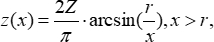(Equation 1)

where Z is the applied displacement, and r is the radius of the rigid cylindrical leg. The incident angle θ1 of the outermost light can be expressed as Equation 2: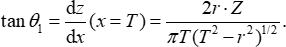(Equation 2)

where T is the radius of the parallel light beam. The refraction angle θ3 of outgoing rays can be expressed as Equation 3 based on Snell's Law2 because tan θ1 tends to be zero: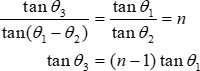(Equation 3)

where n is the refractive index of PDMS. Finally, the relationship between the applied displacement and the shadow diameter change is12,17: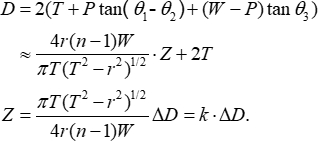(Equation 4)

where P is the thickness of the PDMS piece, W is the working distance between the PDMS surface and the camera, and k is the sensitivity to be calibrated. According to Equation 4, the applied displacement is proportional to the shadow diameter change. Hence, the displacement can be measured by the shadow diameter change after the calibration.

Figure 4 illustrates the diameter fitting process of the shadow images. The original image represented by Figure 4A was converted to the grayscale image and preprocessed by Gaussian filtering and image enhancement, where Figure 4B was obtained. Figure 4C illustrates the sub-pixel edge contour set, which was acquired by the Canny operator. Figure 4D is the least squares circle fit for the circular shadow. Finally, the shadow diameter could be obtained with sub-pixel accuracy.

Figure 5 shows typical shadow images in the process of calibration. The shadow diameter increases as the applied displacement increases. It is observed that the shadow diameter changes symmetrically as the applied displacement increases and decreases.

Figure 6 shows the curve fit of mean values of the changes in shadow diameters due to micro-displacements. The slope of the fitted curve, which denotes the displacement sensitivity, was 10.0 nm/pixel with the fit goodness of 0.9995. Hence, according to Equation 4, the resolution of the measurement system was 10.0 nm when the circle fitting accuracy was 1 pixel. In the measurement range of 5 µm, the maximum deviation was 100.6 nm with a linearity error of 2.01%, which is shown in Figure 7.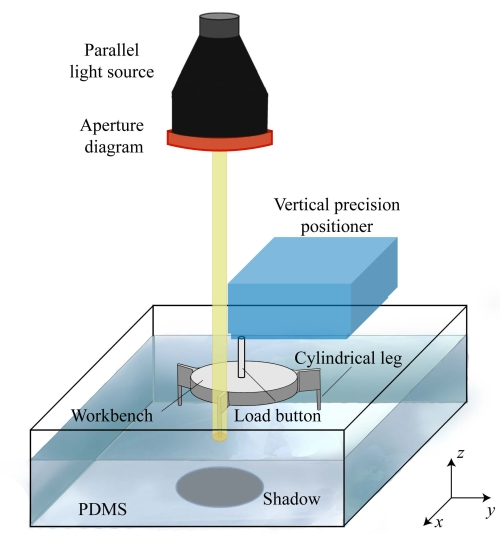Figure 1: Schematic representation of the micro-displacement measurement system. Please click here to view a larger version of this figure.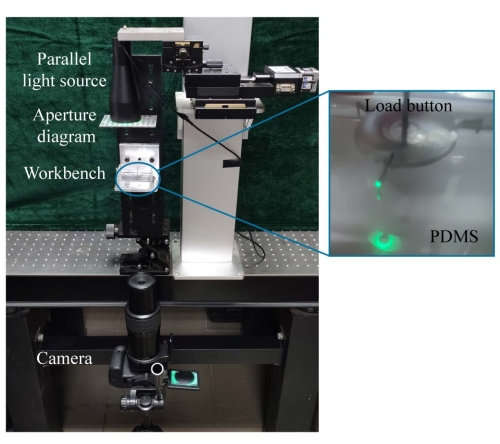Figure 2: Appearance of the micro-displacement measurement system. Inset: Photo of a shadow for display. Please click here to view a larger version of this figure.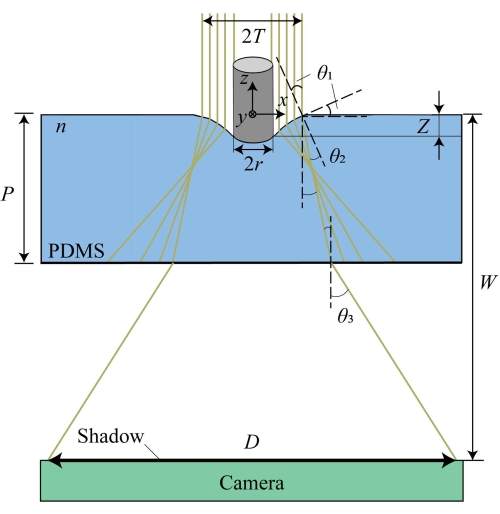Figure 3: Travel path of parallel light beam through the bent surface of the PDMS. Z is the applied displacement. r is the radius of the rigid cylindrical leg. T is the radius of the parallel light beam. n is the refractive index of PDMS. P is the thickness of the PDMS piece. W is the working distance between the PDMS surface and the camera. θ1 and θ2 are the incident angle and the refraction angle of the outermost light. θ3 is the refraction angle of the outgoing rays. D is the shadow diameter. Please click here to view a larger version of this figure.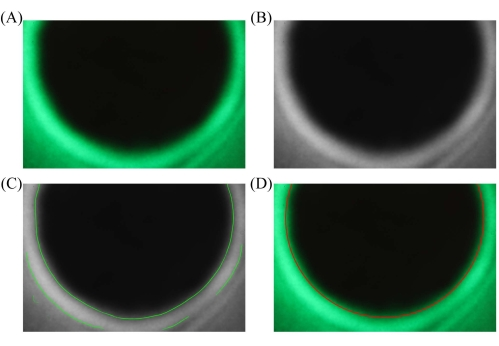Figure 4: Typical processing of the shadow image. (A) RGB shadow image. (B) Preprocessing image. (C) Sub-pixel edge. (D) Least-squares circle fitting. Please click here to view a larger version of this figure.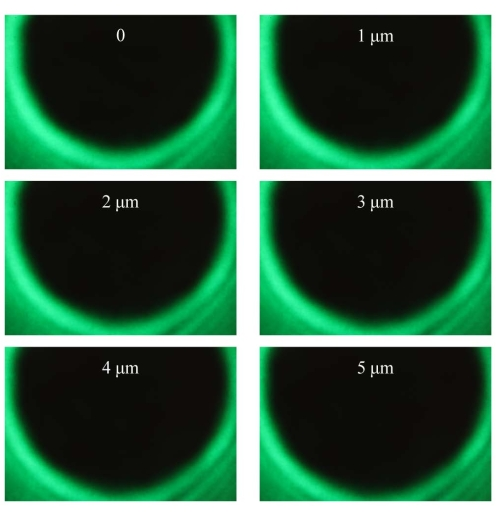Figure 5: Typical shadow images in the process of calibration. The applied displacements are 0 µm, 1 µm, 2 µm, 3 µm, 4 µm, and 5 µm, respectively. Please click here to view a larger version of this figure.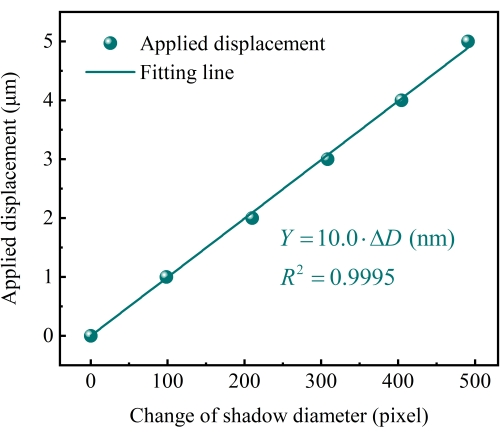Figure 6: Results of micro-displacement. The displacement sensitivity was 10.0 nm/pixel. The goodness of the linear fitting was 0.9995, determined by the least squares method. Please click here to view a larger version of this figure.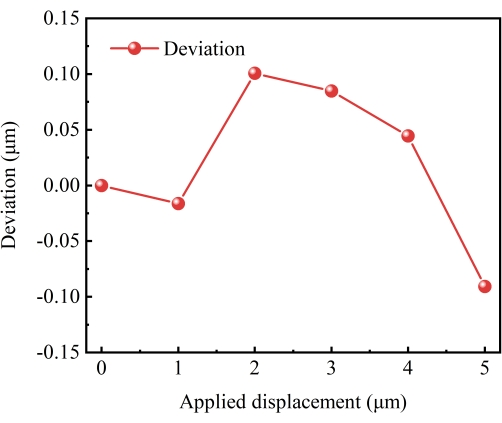Figure 7: Error distribution curve of the displacement calibration. Please click here to view a larger version of this figure.

### Discussion

This protocol proposed a micro-displacement measurement system based on the shadow technique. The displacement calibration is the critical step within the protocol to obtain the displacement sensitivity and the measuring range. The displacement sensitivity can be improved by reducing the diameters of the cylindrical legs and that of the parallel light beam and increasing the working distance based on Equation 4. Furthermore, the pixel size and the resolution of the camera, as well as the accuracy of image processing, are also key to improving displacement sensitivity. The pixel size must be as small as possible to get the smallest change of shadow diameter. The camera resolution should be as big as possible to increase the measuring range. The fitting accuracy of shadow images directly affects the measurement system's performance.

The system based on the shadow method is not suitable for large displacement measurements. The PDMS is a sensitive component that will be damaged due to large deformation. The camera resolution also limits the measuring range. The camera resolution must be high enough to contain all the shadow images. Moreover, the PDMS has a relatively short life of only 2 years at room temperature.

The micro-displacement measurement system has the advantages of simple construction, low cost, high precision, and good linear fitting. Compared with the existing methods5,19, the shadow method can only determine a high-precision displacement measurement with a parallel light source and a camera (such as a mobile phone).

This system shows an advancement in micro-displacement measurements in scientific and industrial fields. For example, it has a great potential in the movement displacement measurement of parts during ultra-precision machining. Furthermore, many mechanical measurements can be first converted into displacement tests through appropriate conversion20, such as the measurement of force, torque, speed, acceleration, temperature, flow, and other parameters.

### Disclosures

The authors have nothing to disclose.

### Acknowledgments

We thank the National Key Research and Development Program of China (No 2021YFC2202702) for funding this work.

### Materials

 Name Company Catalog Number Comments Aperture diaphragm Processed by high precision grinding The diameter of the aperture is 0.7 mm. Camera Canon EOS80D The pixel size and the resolution of the camera are about 3.72 μm and 4000 × 6000, respectively. HALCON MVTec Software GmbH 18.11 MVTec HALCON is the comprehensive standard software for machine vision with an integrated development environment (HDevelop) that is used worldwide. Motorized linear stage Zolix TSA50-C Resolution 0.625 μm Parallel light source Oriental Technology (Shanghai) Co, Ltd. BTPL-50G The peak wavelength is 523 nm. Polydimethylsiloxane (PDMS) Dow Corning Sylgard 184 PDMS is a transparent silicon-based crosslinked polymer. Vacuum pump SHANGHAI LICHEN-BX INSTRUMENT TECHONOLOGY CO.,Ltd 2XZ-6B The pumping rate is 6 L/s.The ultimate vacuum is ≤1 Pa Vertical precision positioner PI P-620.ZCD The resolution is 0.2 nm in the range of 50 μm. Workbench with three rigid cylindrical legs Processed by high precision grinding The diameter of legs is 0.5 mm. The legs are distributed on the trisection points of a circle with a radius of 14 mm

Video Coming Soon
DOIX